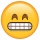Home
IT Knowledge
Inspiration
Languages
EN

# Java - count character occurrences

0 points
Created by:Huzaifa-Ball
415

In this article, we would like to show you how to count occurrences of a character in a String in Java.

Quick solution:

``````String text = "ABC";
char character = 'A';
int result = 0;

for (int i = 0; i < text.length(); i++) {
if (text.charAt(i) == character) {
result++;
}
}

System.out.println(result); // 1``````

## 1. Using simple for loop

In this example, we create simple for loop to iterate through the string and check if each character matches the `character` we want.

``````public class Example {

public static void main(String[] args) {
String text = "ABA";
char character = 'A';
int result = 0;

for (int i = 0; i < text.length(); i++) {
if (text.charAt(i) == character) {
result++;
}
}

System.out.println("result = " + result); // 2
}
}``````

Output:

``result = 2``

## 2. Using String `replace()`

In this example, we use String `replace()` method with string length subtraction to count the occurrences of the `A` character in the `text`.

``````public class Example {

public static void main(String[] args) {
String text = "ABA";
int result = text.length() - text.replace("A", "").length();

System.out.println("result = " + result);  // 2
}
}``````

Output:

``result = 2``

## 3. Using String `replaceAll()`

In this example, we use String `replace()` method with `[^A]` regex to count the occurrences of the `A` character in the `text`.

Regex explanation:

• `[^ ]` is the negation operator, negated set (matches any character that is not in the set),
• `[^A]` matches any character except A.

Practical example:

``````public class Example {

public static void main(String[] args) {
String text = "ABA";
int result = text.replaceAll("[^A]", "").length();

System.out.println("result = " + result);  // 2
}
}``````

Output:

``result = 2``

## 4. Using String `split()`

In this example, we use String `split()` method with string `length()` to count the occurrences of the `A` character in the `text`.

``````public class Example {

public static void main(String[] args) {
String text = "ABA";
int result = text.split("A", -1).length - 1;

System.out.println("result = " + result);  // 2
}
}``````

Output:

``result = 2``